# The open loop gain of a control system is given by: G(S) (s +2)3 find the...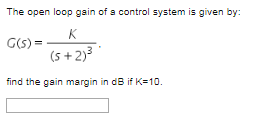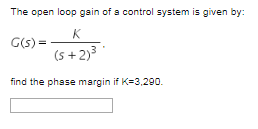The open loop gain of a control system is given by: G(S) (s +2)3 find the gsin margin in dB if K-10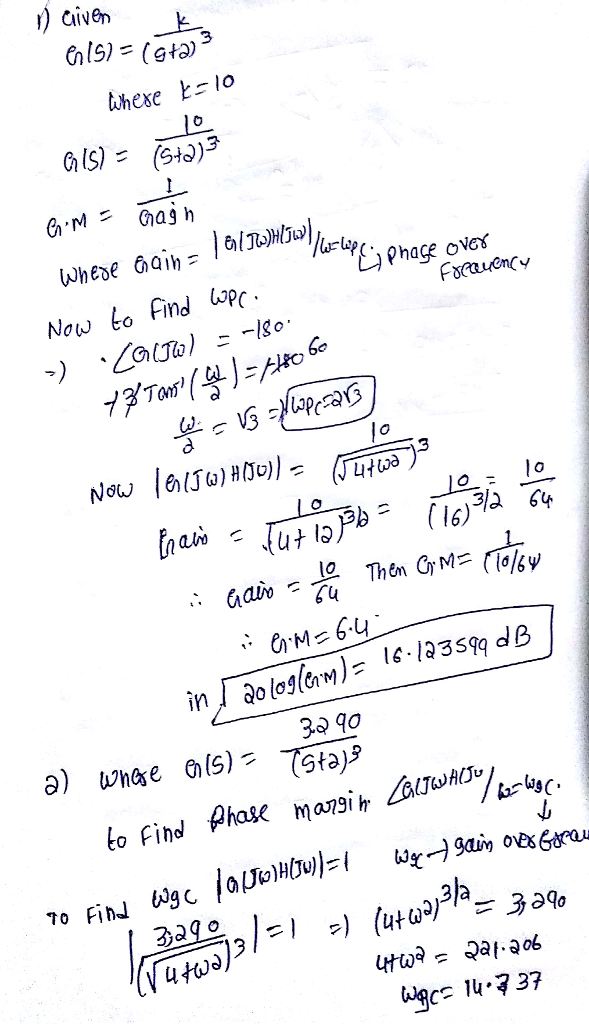#### Earn Coin

Coins can be redeemed for fabulous gifts.

Similar Homework Help Questions
• ### Find a & b Figure 1 shows a closed-loop control system in which G(S)-40/1 (S+2) (S+3)],...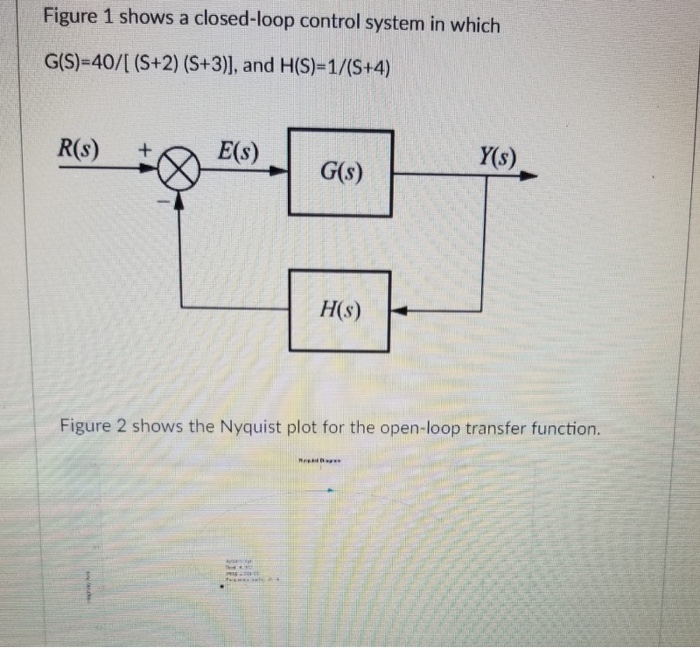Find a & b Figure 1 shows a closed-loop control system in which G(S)-40/1 (S+2) (S+3)], and H(S)-1/(S+4) Y(s) H(s) Figure 2 shows the Nyquist plot for the open-loop transfer function. System: sys Real: -0.187 Imag: 2.56e-05 Frequency: (rad/s): -5.16 Using the Nyquist criterion a) Find out the gain margin expressed in dB. Is the system stable or unstable? (25 points) b) What is the value of the gain expressed in dB that makes the system marginally stable? (25 points)

• ### Figure 1 shows a closed-loop control system in which G(S)=40/[ (S+2) (S+3)], and H(S)=1/(S+4) R(S) E(S)...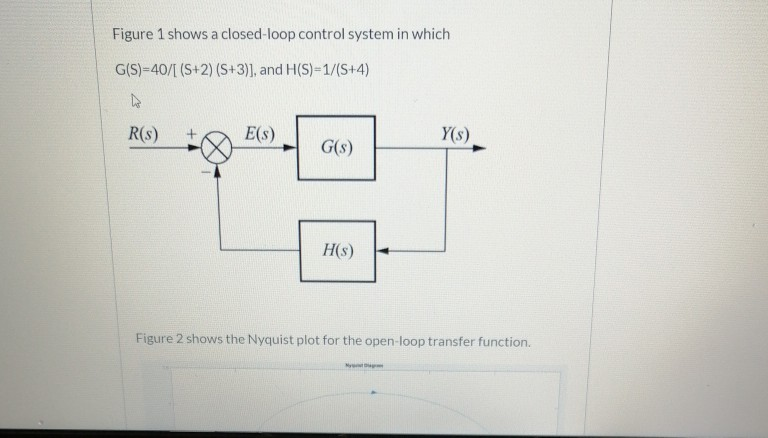Figure 1 shows a closed-loop control system in which G(S)=40/[ (S+2) (S+3)], and H(S)=1/(S+4) R(S) E(S) Y(s) G(S) HS) Figure 2 shows the Nyquist plot for the open-loop transfer function. Figure 2 shows the Nyquist plot for the open-loop transfer function System: sys Real: -0.187 Imag: 2.56e-05 Frequency: (rad/s): -5.16 Using the Nyquist criterion: a) Find out the gain margin expressed in dB. Is the system stable or unstable? (25 points) b) What is the value of the gain expressed...

• ### A unity gain negative feedback system has an open-loop transfer function given by 4. s) =...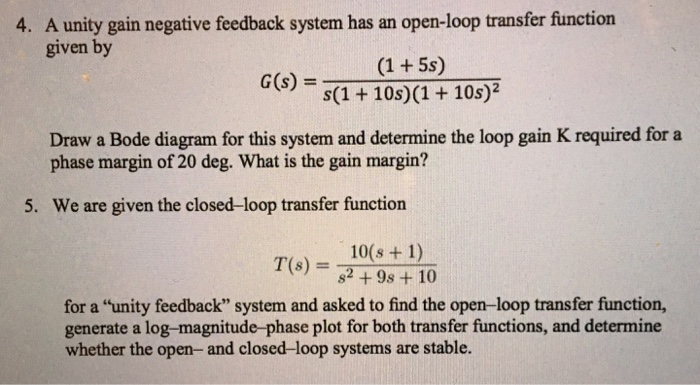A unity gain negative feedback system has an open-loop transfer function given by 4. s) = s(1 + 10s)(1 + 10s)? Draw a Bode diagram for this system and determine the loop gain K required for a phase margin of 20 deg. What is the gain margin? 5. We are given the closed-loop transfer function 10(s + 1) T(s) = 82+98+10 for a "unity feedback" system and asked to find the open-loop transfer function, generate a log-magnitude-phase plot for both...

• ### Figure 1 shows a closed-loop control system in which G(S)=40/[ (S+2) (S+3)], and H(S)=1/(S+4) R(3) +...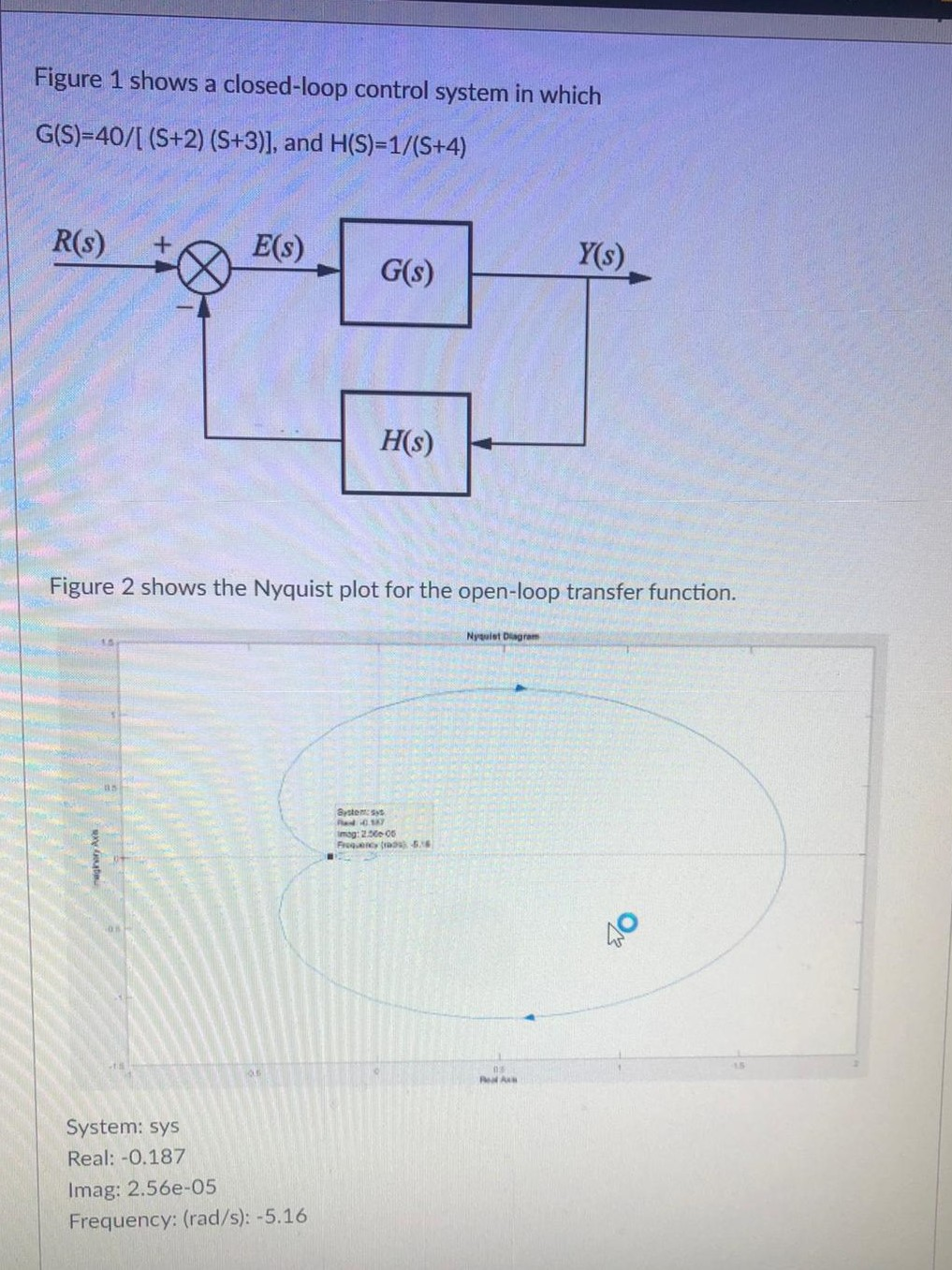Figure 1 shows a closed-loop control system in which G(S)=40/[ (S+2) (S+3)], and H(S)=1/(S+4) R(3) + E(S) Y() G(s) H(S) Figure 2 shows the Nyquist plot for the open-loop transfer function. Nywist Diagram Systems imag: 2.5606 FC-56 THVL AM On RAH System: sys Real: -0.187 Imag: 2.56e-05 Frequency: (rad/s): -5.16 Figure 2 shows the Nyquist plot for the open-loop transfer function. Nyulat Diagram 05 Systems imag: 250 os ghar Axle 5.10 05 System: sys Real: -0.187 Imag: 2.56e-05 Frequency: (rad/s):...

• ### Figure 1 shows a closed-loop control system in which G(S)=40/[ (S+2) (S+3)], and H(S)=1/(S+4) R(3) E(s)...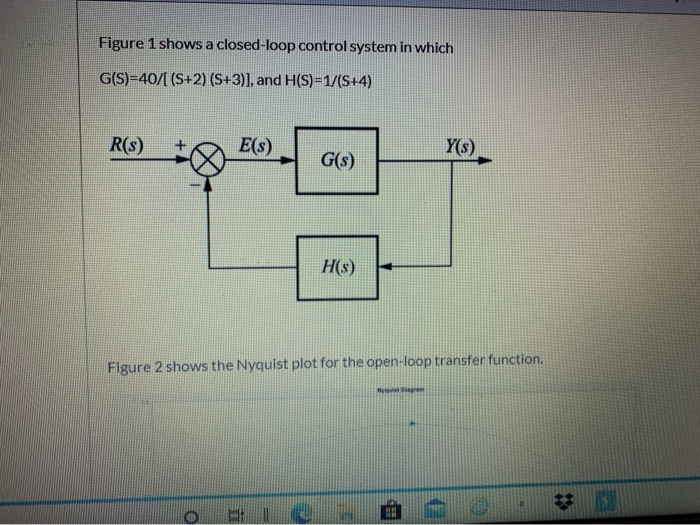Figure 1 shows a closed-loop control system in which G(S)=40/[ (S+2) (S+3)], and H(S)=1/(S+4) R(3) E(s) Y(s) G(s) H(s) Figure 2 shows the Nyquist plot for the open-loop transfer function. NON BH Figure 2 shows the Nyquist plot for the open-loop transfer function. NOM & NON System: sys Real: -0.187 Imag: 2.56e-05 Frequency: (rad/s): -5.16 structure.com/courses/68755/quizzes/411964/take S 2 NA System: sys Real: -0.187 Imag: 2.56e-05 Frequency: (rad/s): -5.16 Using the Nyquist criterion: a) Find out the gain margin expressed in...

• ### Figure 1 shows a closed-loop control system in which G(S)-40/1 (5+2) (5+3)], and H(S)-1/15+4) R(s) E(S)...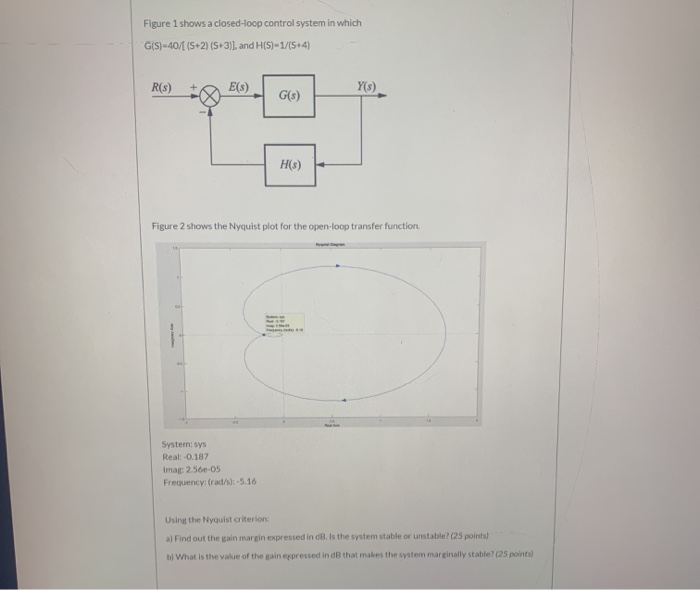Figure 1 shows a closed-loop control system in which G(S)-40/1 (5+2) (5+3)], and H(S)-1/15+4) R(s) E(S) Y(5) G(s) H(s) Figure 2 shows the Nyquist plot for the open-loop transfer function. Systemsys Real: -0.187 Imag: 2.56e-05 Frequency: (rad/s): -5.16 Using the Nyquist criterion a) Find out the gain margin expressed in dB. Is the system stable or unstable? (25 points) b) What is the value of the gain expressed in dB that makes the system marginally stable?(25 points)

• ### 6) The open loop transfer function of a control system is 10-011. Ata frequency of 1...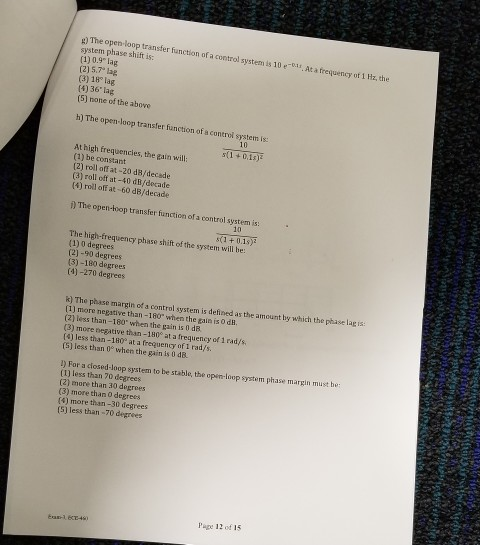6) The open loop transfer function of a control system is 10-011. Ata frequency of 1 Hz, the system phase shift is: (1) 0.9 kg (2) 5.7" tag (3) 18 (6) 36"lag (5) none of the above h) The open-loop transfer function of control system is 10 At high frequencies, the gain will: (1) be constant (2) roll off at -20 d/decade (3) roll off at -40 dB/decade (6) roll off at 60 dB/decade The open-loop transfer function of a...

• ### Question 6 The open-loop transfer function G(s) of a control system is given as G(8)- s(s+2)(s +5...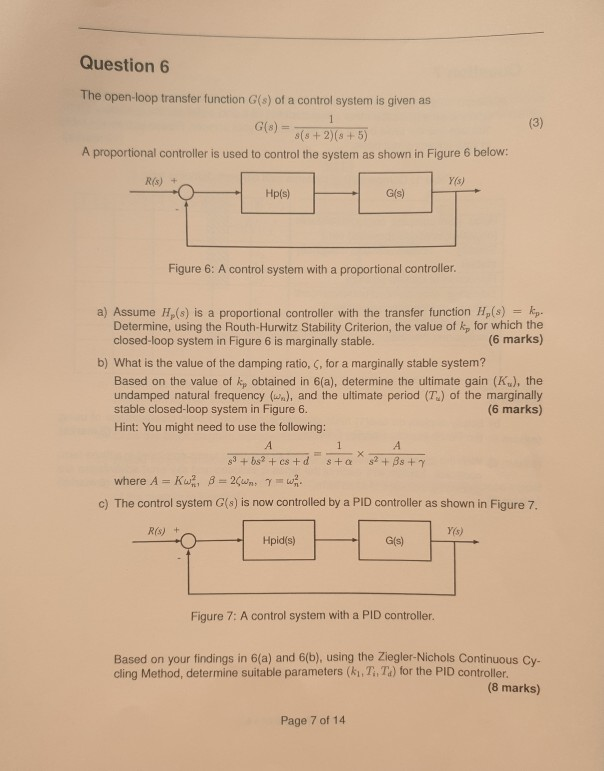Question 6 The open-loop transfer function G(s) of a control system is given as G(8)- s(s+2)(s +5) A proportional controller is used to control the system as shown in Figure 6 below: Y(s) R(s) + G(s) Figure 6: A control system with a proportional controller a) Assume Hp(s) is a proportional controller with the transfer function H,(s) kp. Determine, using the Routh-Hurwitz Stability Criterion, the value of kp for which the closed-loop system in Figure 6 is marginally stable. (6...

• ### Please solve clearly and step by step. nity-feedback open-loop transfer function is B-7-28. Consider a u control system...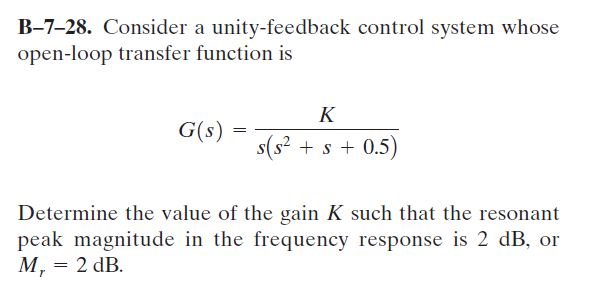Please solve clearly and step by step. nity-feedback open-loop transfer function is B-7-28. Consider a u control system whose G(s) s(s2+s +0.5) Determine the value of the gain K such that the resonant pcak magnitude in te frequcncy response is 2 dB, or M, 2 dB. nity-feedback open-loop transfer function is B-7-28. Consider a u control system whose G(s) s(s2+s +0.5) Determine the value of the gain K such that the resonant pcak magnitude in te frequcncy response is 2...

• ### Question ④ (20 marks) Consider a control system shown in Fig. I has an open loop TF-G(s) H (s)--( A-Prove that the gain margin-infinite db at infinite rad/sec. and the phase margin 62.1 degrees at...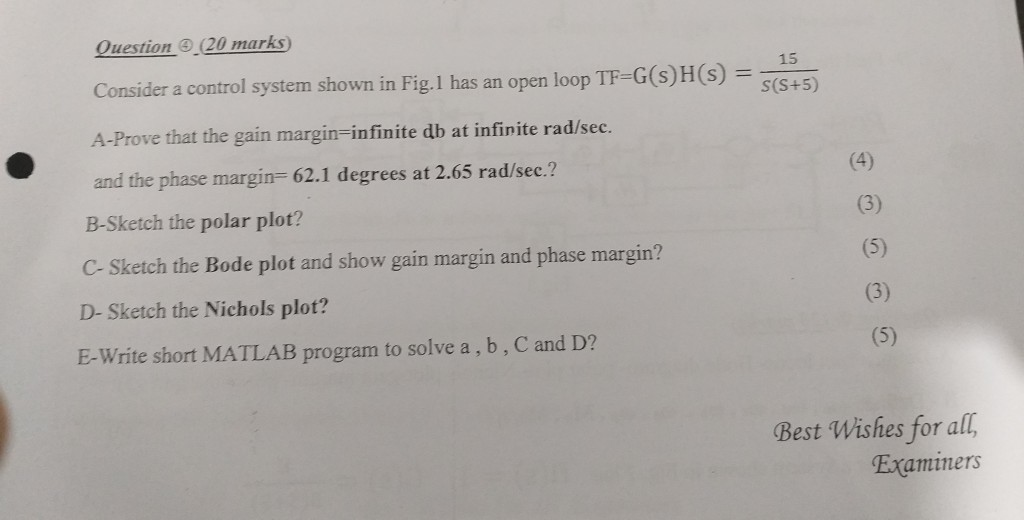Question ④ (20 marks) Consider a control system shown in Fig. I has an open loop TF-G(s) H (s)--( A-Prove that the gain margin-infinite db at infinite rad/sec. and the phase margin 62.1 degrees at 2.65 rad/sec.? B-Sketch the polar plot? 15 S(S+5) C- Sketch the Bode plot and show gain margin and phase margin? D-Sketch the Nichols plot? E-Write short MATLAB program to solve a, b, C and D? Best Wishes for all, Examiners Question ④ (20 marks) Consider...